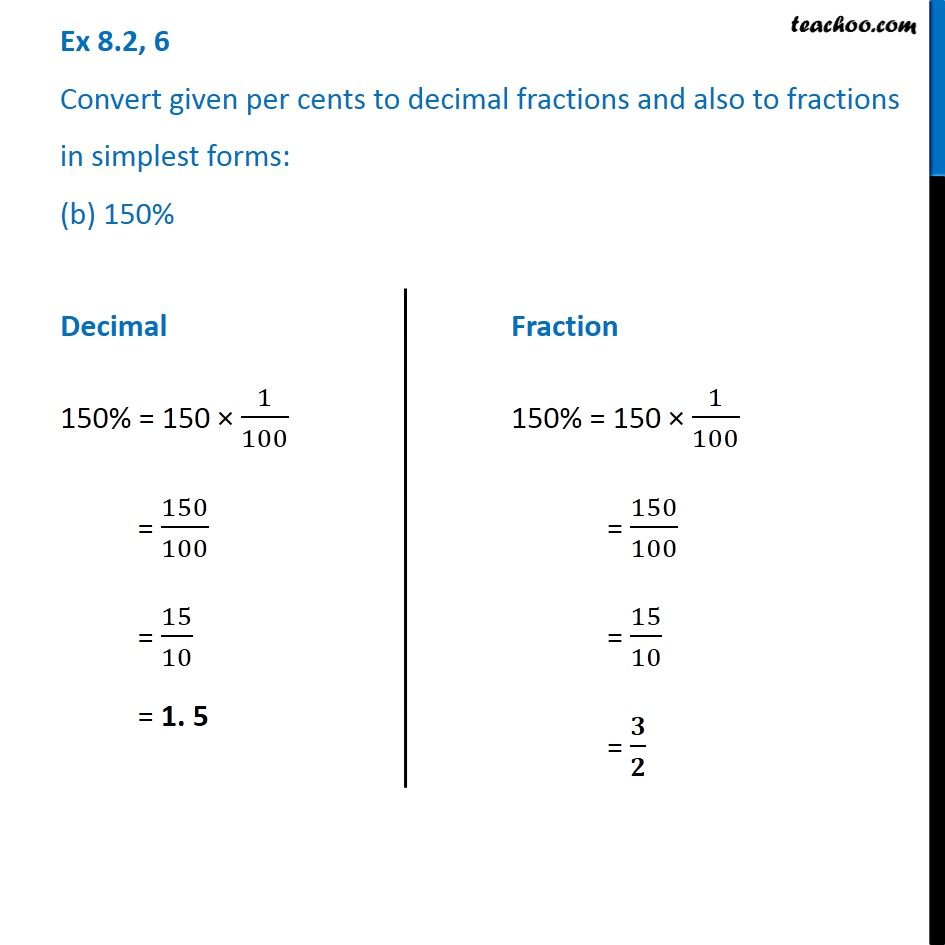1. Chapter 8 Class 7 Comparing Quantities
2. Serial order wise
3. Ex 8.2

Transcript

Ex 8.2, 6 Convert given per cents to decimal fractions and also to fractions in simplest forms: (b) 150% Decimal 150% = 150 × 1/100 = 150/100 = 15/10 = 1. 5 Fraction 150% = 150 × 1/100 = 150/100 = 15/10 = 𝟑/𝟐